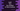# Swift program to find the smallest of three numbers## Swift program to find the smallest of three numbers:

We can find the smallest of three numbers in swift easily. We can either compare each one of these values with one another or we can use the min() function. In this post, we will learn how to find the smallest of three numbers in Swift.

In this post, we will learn how to find the smallest of three numbers in different ways.

## Method 1: Find the smallest of three numbers by comparing with one another:

Below program finds the smallest of three numbers by comparing each with one another:

``````func findMin(x: Int, y: Int) -> Int{
return x < y ? x : y
}

func findMinThree(first: Int, second: Int, third: Int) -> Int{
let minFirstTwo = findMin(x: first, y: second)
let minLastTwo = findMin(x: second, y: third)

return findMin(x: minFirstTwo, y: minLastTwo)
}

print(findMinThree(first: 1, second: 2, third: 3))
print(findMinThree(first: 1, second: 3, third: 2))
print(findMinThree(first: 3, second: 1, third: 2))
print(findMinThree(first: 3, second: 2, third: 1))
print(findMinThree(first: 3, second: 3, third: 3))``````

Here,

• findMin method is used to find the smaller value among two numbers.
• findMinThree method is used to find the smallest value among three numbers.
• We are calculating the smaller value of the first and the second number and this value is stored in minFirstTwo. Similarly, the smaller value of the second and third number is stored in minLastTwo.
• It returns the smaller value of minFirstTwo and minLastTwo, which is the smallest number.

If you run this program, it will print the below output:

``````1
1
1
1
3``````

## Method 2: Find the smallest of three numbers by using min():

We can also find the smallest of three numbers by using the min() function. This function can find the smaller value of two numbers:

``````func findMinThree(first: Int, second: Int, third: Int) -> Int{
let minFirstTwo = min(first, second)
let minLastTwo = min(second, third)

return min(minFirstTwo, minLastTwo)
}

print(findMinThree(first: 1, second: 2, third: 3))
print(findMinThree(first: 1, second: 3, third: 2))
print(findMinThree(first: 3, second: 1, third: 2))
print(findMinThree(first: 3, second: 2, third: 1))
print(findMinThree(first: 3, second: 3, third: 3))``````

It makes the program smaller. If you run this program, it will print similar output.

## Method 3: By using min(), without any extra method:

We can also pass three numbers to min() function. It will give similar output:

``````print(min(1, 2, 3))
print(min(1, 3, 2))
print(min(3, 1, 2))
print(min(3, 2, 1))
print(min(3, 3, 3))``````

It will print similar output.